# The following MINITAB output presents the results of a hypothesis test for the differenceP1 - P2 between two population proportions. Some of the numbers are missing. Fill in thenumbers for (a) through (d).Test and CI for Two ProportionsSample p(a)Sampleх1011532(b)900.544444Difference = p (1) - (2)Estimate for difference: 0.11568695% CI for difference: (-0.0116695, 0.243042)Test for difference = 0 (vs not = 0): Z = (c) P-Value = (d)%3D%3D

Question
1 views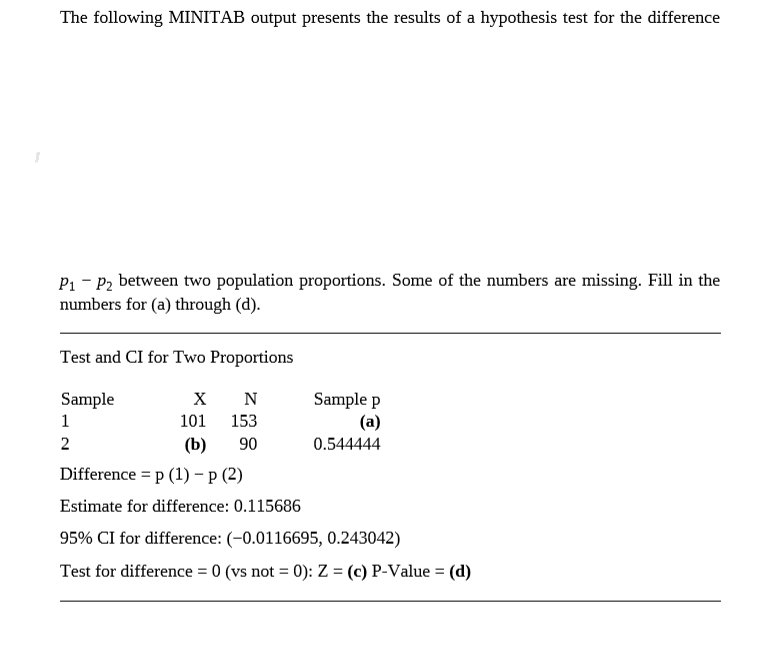help_outlineImage TranscriptioncloseThe following MINITAB output presents the results of a hypothesis test for the difference P1 - P2 between two population proportions. Some of the numbers are missing. Fill in the numbers for (a) through (d). Test and CI for Two Proportions Sample p (a) Sample х 101 153 2 (b) 90 0.544444 Difference = p (1) - (2) Estimate for difference: 0.115686 95% CI for difference: (-0.0116695, 0.243042) Test for difference = 0 (vs not = 0): Z = (c) P-Value = (d) %3D %3D fullscreen
check_circle

Step 1

The values missed in the table will be obtained as given below:

(a)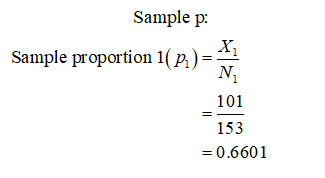Step 2

(b)

The number of observation in Sample 2: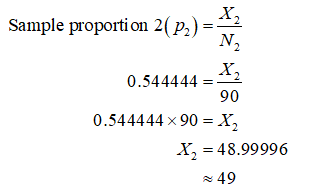Step 3

(c)

Standard error of the difference between two proportions (p-hat 1 – p-hat 2):

Here, p-hat1 = 0.6601, n1 = 153, p-hat 2 = 0.5444, n2 = 90.

Denote the population proportion as p.

The standard error of the difference between two proportions (p-hat 1 – p-hat 2)is obtained from the calculation given below: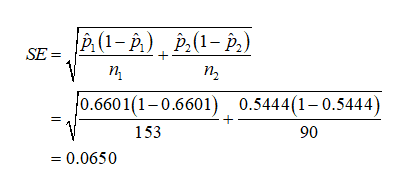The test statistic is given by,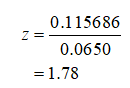...

### Want to see the full answer?

See Solution

#### Want to see this answer and more?

Solutions are written by subject experts who are available 24/7. Questions are typically answered within 1 hour.*

See Solution
*Response times may vary by subject and question.
Tagged in